Related Articles
Class 9 NCERT Solutions- Chapter 7 Triangles – Exercise 7.1
• Last Updated : 28 Dec, 2020

### Question 1. In quadrilateral ACBDAC = AD and AB bisects ∠ A (see Fig. 7.16). Show that ∆ ABC ≅ ∆ ABD What can you say about BC and BD?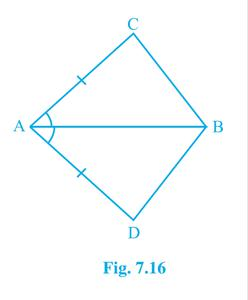Solution:

Given that AC and AD are equal

i.e. AC = AD and the line AB bisects ∠A.

Considering the two triangles ΔABC and ΔABD,

Where,

AC = AD { As given}………………………………………… (i)

∠CAB = ∠DAB ( As AB  bisects of ∠A)……………. (ii)

AB { Common side of both the triangle} …….. …(iii)

From above three equation both the triangle satisfies “SAS” congruency criterion

So, ΔABC ≅ ΔABD.

Also,

BC and BD will be of equal lengths as they are corresponding parts of congruent triangles(CPCT).

So BC = BD.

### Question 2. ABCD is a quadrilateral in which AD = BC and ∠ DAB = ∠ CBA (see Fig. 7.17). Prove that

(i) ∆ ABD ≅ ∆ BAC

(ii) BD = AC

(iii) ∠ ABD = ∠ BAC.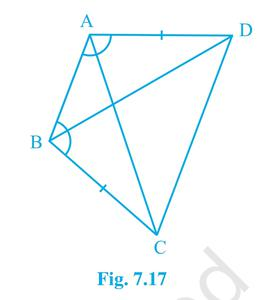Solution:

(i) Given that AD = BC,

And ∠ DAB = ∠ CBA.

Considering two triangles ΔABD and ΔBAC.

Where,

AD = BC { As given }………………………………………….. (i)

∠ DAB = ∠ CBA { As given also}……………………….. (ii)

AB  {Common side of both the triangle)…………. (iii)

From above three equation two triangles ABD and BAC satisfies “SAS” congruency criterion

So, ΔABD ≅ ΔBAC

(ii) Also,

BD and AC  will be equal as they are corresponding parts of congruent triangles(CPCT).

So BD = AC

(iii) Similarly,

∠ABD and ∠BAC will be equal as they are corresponding parts of congruent triangles(CPCT).

So,

∠ABD = ∠BAC.

### Question 3. AD and BC are equal perpendiculars to a line segment AB (see Fig. 7.18). Show that CD bisects AB.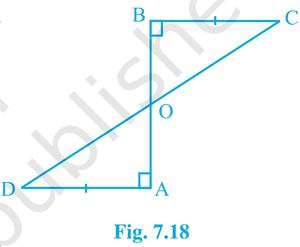Solution:

Given that AD and BC are two equal perpendiculars to a line segment AB

Considering two triangles ΔAOD and ΔBOC

Where,

∠ AOD = ∠ BOC {Vertically opposite angles}………………. (i)

∠ OAD = ∠ OBC {Given that they are perpendiculars}…. (ii)

AD = BC {As given}………………………………………………… (iii)

From above three equation both the triangle satisfies “AAS” congruency criterion

So, ΔAOD ≅ ΔBOC

AO and BO will be equal as they are corresponding parts of congruent triangles(CPCT).

So, AO = BO

Hence, CD bisects AB at O.

### Question 4. l and m are two parallel lines intersected by another pair of parallel lines p and q (see Fig. 7.19). Show that ∆ ABC ≅ ∆ CDA.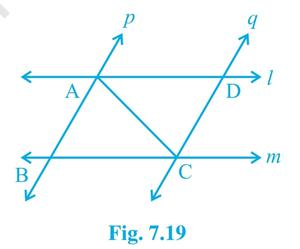Solution:

Given that l and m are two parallel lines p and q are another pair of parallel lines

Considering two triangles ΔABC and ΔCDA

Where,

∠ BCA = ∠DAC  {Alternate interior angles}…. (i)

∠ BAC = ∠ DCA {Alternate interior angles}…. (ii)

AC  {Common side of two triangles}………….(iii)

From above three equation both the triangle satisfies “ASA” congruency criterion

So, ΔABC ≅ ΔCDA

### Question 5. Line l is the bisector of an angle ∠ A and B is any point on l. BP and BQ are perpendiculars from B to the arms of ∠A (see Fig. 7.20). Show that:

(i) ∆ APB ≅ ∆ AQB

(ii) BP = BQ or B is equidistant from the arms of ∠ A.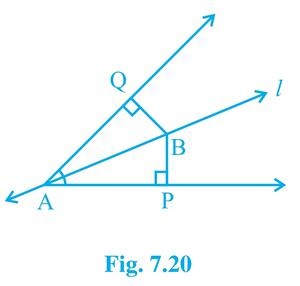Solution:

Given that, Line l is the bisector of an angle ∠ A and B

BP and BQ are perpendiculars from angle A.

Considering two triangles ΔAPB and ΔAQB

Where,

∠ APB = ∠ AQB { Two right angles as given }…… (i)

∠BAP = ∠BAQ (As line l  bisects  angle A }……… (ii)

AB  { Common sides of both the triangle }……… (iii)

From above three equation both the triangle satisfies “AAS” congruency criterion

So, ΔAPB≅ ΔAQB.

(ii) Also we can say BP and BQ are equal as they are corresponding parts of congruent triangles(CPCT).

So, BP = BQ

### Question 6. In Fig. 7.21, AC = AE, AB = AD and ∠ BAD = ∠ EAC. Show that BC = DE.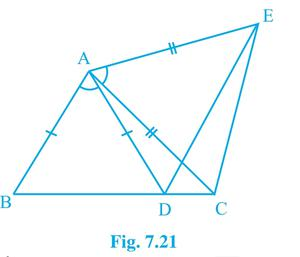Solution:

Given that AC = AE, AB = AD

And ∠BAD = ∠EAC

As given that ∠BAD = ∠EAC

Adding ∠DAC on both the sides

We get,

∠BAD + ∠DAC = ∠EAC + ∠ DAC

∠BAC = ∠EAD

Considering two triangles ΔABC and ΔADE

Where,

AC = AE  { As given }…………………… (i)

∠BAC = ∠EAD { Hence proven }…….. (ii)

AB = AD {As also given }……………….. (iii)

From above three equation both the triangle satisfies “SAS” congruency criterion

So, ΔABC ≅ ΔADE

(ii) Also we can say BC and  DE are equal as they are corresponding parts of congruent triangles(CPCT).

So that BC = DE.

### Question 7. AB is a line segment and P is its mid-point. D and E are points on the same side of AB such that ∠ BAD = ∠ ABE and ∠ EPA = ∠ DPB (see Fig. 7.22). Show that:

(i) ∆ DAP ≅ ∆ EBP

(ii) AD = BE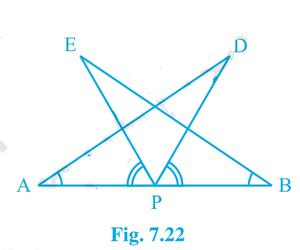Solution:

Given that P is the mid-point of line  AB, So AP = BP

Also, ∠ BAD = ∠ ABE and ∠ EPA = ∠ DPB

Now adding ∠DPE on both the sides of two equal angle ∠ EPA = ∠ DPB

∠ EPA + ∠ DPE = ∠ DPB + ∠DPE

Which implies that two angles ∠ DPA = ∠ EPB

Considering two triangles ∆ DAP and ∆ EBP

∠ DPA = ∠ EPB { Hence proven }…… (i)

AP = BP { Hence Given }……………… (ii)

∠ BAD = ∠ ABE { As given }…………..(iii)

From above three equation both the triangle satisfies “ASA” congruency criterion

So, ΔDAP ≅ ΔEBP

(ii) Also we can say AD and BE are equal as they are corresponding parts of congruent triangles(CPCT).

So that, AD = BE

### Question 8. In right triangle ABC, right angled at C, M is the mid-point of hypotenuse AB. C is joined to M and produced to a point D such that DM = CM. Point D is joined to point B (see Fig. 7.23). Show that:

(i) ∆ AMC ≅ ∆ BMD

(ii) ∠ DBC is a right angle.

(iii) ∆ DBC ≅ ∆ ACB

(iv) CM =  1/2 AB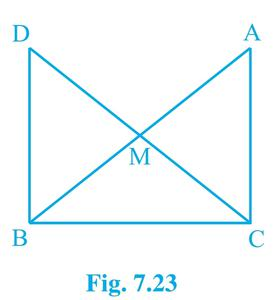Solution:

Given that M is the mid-point of  AB

So AM = BM

∠ ACB = 90°

and DM = CM

(i) Considering two triangles ΔAMC and ΔBMD:

AM = BM { As given }………………………………………. (i)

∠ CMA = ∠ DMB { Vertically opposite angles }…. (ii)

CM = DM { As given also}……………………………….. (iii)

From above three equation both the triangle satisfies “SAS” congruency criterion

So, ΔAMC ≅ ΔBMD

(ii) From above congruency we can say

∠ ACD = ∠ BDC

Also alternate interior angles of two parallel lines AC and DB.

Since sum of two co-interiors angles results to 180°.

So, ∠ ACB + ∠ DBC = 180°

∠ DBC = 180° – ∠ ACB

∠ DBC = 90° { As ∠ACB =90° }

(iii) In ΔDBC and ΔACB,

BC  { Common side of both the triangle }……. (i)

∠ ACB = ∠ DBC { As both are right angles }….(ii)

DB = AC (by CPCT)………………………………….. (iii)

From above three equation both the triangle satisfies “SAS” congruency criterion

So, ΔDBC ≅ ΔACB

(iv) As M is the mid point so we can say

DM = CM = AM = BM

Also we can say that AB = CD ( By CPCT )

As M is the mid point of CD we can write

CM + DM = AB

Hence, CM + CM = AB  (As DM = CM )

Hence, CM = (½) AB

Attention reader! Don’t stop learning now. Get hold of all the important DSA concepts with the DSA Self Paced Course at a student-friendly price and become industry ready.

My Personal Notes arrow_drop_up